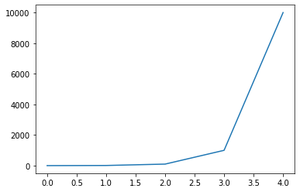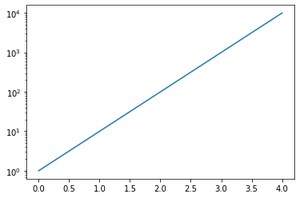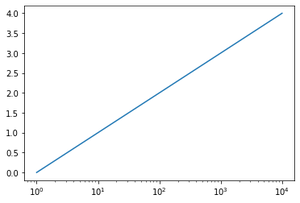Related Articles

# How to Plot Logarithmic Axes in Matplotlib?

• Difficulty Level : Medium
• Last Updated : 21 Jan, 2021

Axes’ in all plots using Matplotlib are linear by default, yscale() and xscale() method of the matplotlib.pyplot library can be used to change the y-axis or x-axis scale to logarithmic respectively.

The method yscale() or xscale() takes a single value as a parameter which is the type of conversion of the scale, to convert axes to logarithmic scale we pass the “log” keyword or the matplotlib.scale. LogScale class to the yscale or xscale method.

xscale method syntax:

Syntax : matplotlib.pyplot.xscale(value, **kwargs)

Parameters:

• Value = { “linear”, “log”, “symlog”, “logit”, … }
• **kwargs = Different keyword arguments are accepted, depending on the scale (matplotlib.scale.LinearScale, LogScale, SymmetricalLogScale, LogitScale)

Returns : Converts the x-axes to the given scale type. (Here we use the “log” scale type)

yscale method syntax:

Syntax: matplotlib.pyplot.yscale(value, **kwargs)

Parameters:

• value = { “linear”, “log”, “symlog”, “logit”, … }
• **kwargs = Different keyword arguments are accepted, depending on the scale (matplotlib.scale.LinearScale, LogScale, SymmetricalLogScale, LogitScale)

Returns : Converts the y-axes to the given scale type. (Here we use the “log” scale type)

Given below are the implementation for converting the y-axis and x-axis to logarithmic scale respectively.

Example 1: Without Logarithmic Axes.

## Python3

 `import` `matplotlib.pyplot as plt` `# exponential function y = 10^x``data ``=` `[``10``*``*``i ``for` `i ``in` `range``(``5``)]` `plt.plot(data)`

Output:Example 2: y-axis Logarithmic Scale.

## Python3

 `import` `matplotlib.pyplot as plt` `# exponential function y = 10^x``data ``=` `[``10``*``*``i ``for` `i ``in` `range``(``5``)]` `# convert y-axis to Logarithmic scale``plt.yscale(``"log"``)` `plt.plot(data)`

Output:Example 3: x-axis Logarithmic Scale.

## Python3

 `import` `matplotlib.pyplot as plt` `# exponential function x = 10^y``datax ``=` `[ ``10``*``*``i ``for` `i ``in` `range``(``5``)]``datay ``=` `[ i ``for` `i ``in` `range``(``5``)]` `#convert x-axis to Logarithmic scale``plt.xscale(``"log"``)` `plt.plot(datax,datay)`

Output:Attention geek! Strengthen your foundations with the Python Programming Foundation Course and learn the basics.

To begin with, your interview preparations Enhance your Data Structures concepts with the Python DS Course. And to begin with your Machine Learning Journey, join the Machine Learning – Basic Level Course

My Personal Notes arrow_drop_up